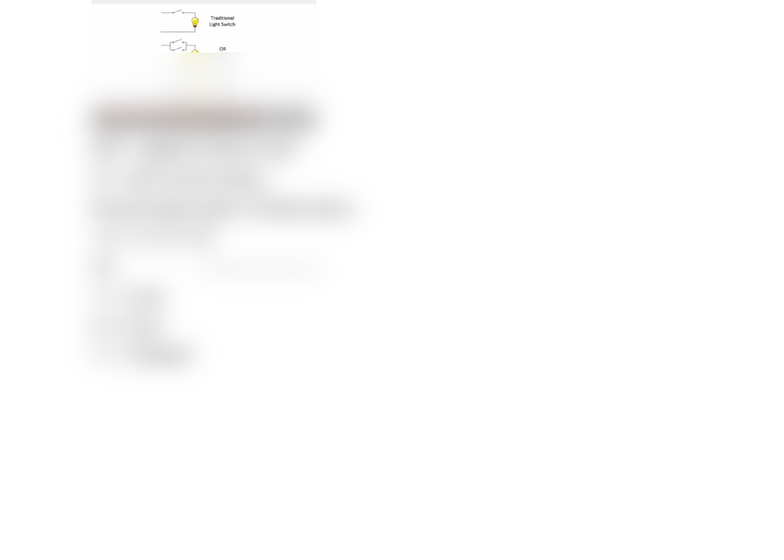Class Notes (1,100,000)
CA (650,000)
UW (20,000)
CS (1,000)
CS100 (100)
Lecture 11

# CS100 Lecture Notes - Lecture 11: Discretization, Extended Ascii

Department
Computer Science
Course Code
CS100
Professor
Alexandra Boutros
Lecture
11

This preview shows half of the first page. to view the full 2 pages of the document.Module 6
6.1
Discrete (Digital) vs Continuous (Analog)
Discrete no in-between numbers
Continuous infinite amount of numbers (between numbers are infinite)
Discretization (digitization) make a continuous info discrete to reduce amount of info given
and make it more convenient
Computers have a natural tendency to digitize (discrete) everything
6.2 bit of memory
In a computer, lets assume a light bulb, 0 = off, 1 = on
Billions of switches in the computer, they are for logic and memory
Bit binary digit, byte = 8 bits
NOT opposite of what we want
6.3 how to count in binary
Decimal number system (10 based system)
^ 0 1 2 3 4 5 6 7 8 9
207 ------------------------
7 = 7 ones
0 = 0 tens”
2 = 2 hundreds”